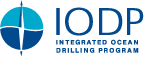IODP Proceedings    Volume contents     Searchdoi:10.2204/iodp.proc.333.205.2017

## Results

Tables T2 and T3 show the peak area values (total counts) for common minerals in the clay-size fraction, segregated by lithologic unit. Tables T2 and T3 also include the values of mineral abundance (wt%) calculated with SVD normalization factors and weighted peak area percentages using Biscaye (1965) factors. Those SVD values are rounded to the nearest 1 wt% in acknowledgment of the statistical disadvantages of using three sets of SVD normalization factors and two diffractometers.

### Site C0011

The relative abundance of clay-size smectite within the hemipelagic/pyroclastic facies (Unit I) ranges from 12 to 68 wt% (Fig. F4) with a mean value (µ) of 40.8 wt% and a standard deviation (s) of 11.5. The depth distribution defines a general trend of steadily increasing smectite content downsection. The amount of illite in the clay-size fraction ranges from 23 to 49 wt% (µ = 36.4 wt%; s = 5.7), and we recognize a general trend of decreasing illite content downsection. Percentages of chlorite range from 5 to 29 wt% (µ = 15.5 wt%; s = 4.8). Percentages of clay-size kaolinite and quartz average 3.6 wt% (s = 3.2) and 3.7 wt% (s = 4.0), respectively.

Samples from the upper intervals of Unit II (volcanic turbidite facies) are similar in clay composition to those near the base of Unit I (Fig. F4). Percentages of clay-size smectite range from 37 to 97 wt%, and illite varies from 3 to 43 wt%. Interested readers should refer to Underwood and Guo (2013) to see continuations of those trends farther downsection.

Figure F5 illustrates how the abundance of smectite within the bulk sediment changes as a function of depth. Within Unit I, the values range from 7 to 48 wt% (µ = 27.1 wt%; s = 8.4). Comparable values within lithologic Unit II range from 29 to 55 wt%, continuing the general trend of increasing percentages of smectite with increasing depth and age.

Illite crystallinity (Kübler) index values within Unit I range from 0.84Δ°2θ to 0.27Δ°2θ with an average of 0.43Δ°2θ. We consider such values as basic indicators of generic conditions within detrital source areas. As a qualitative frame of reference, the boundary between advanced diagenesis and anchizone metamorphism is set at 0.52Δ°2θ, and the achizone/epizone boundary (incipient greenschist facies) coincides with 0.32Δ°2θ (Warr and Mählmann, 2015). We see no systematic changes in illite crystallinity with depth. Similarly, the expandability of smectite and I/S mixed-layer clay ranges from 49% to 91% (µ = 66%), with no systematic changes as a function of depth.

### Site C0012

The relative abundance of clay-size smectite within Unit I ranges from 22 to 69 wt% (Fig. F6). As at Site C0011, results define a general trend in which smectite content increases downsection, although with more scatter and a higher mean (µ = 50.0 wt%; s = 13.5). Values for illite range from 18 to 46 wt% (µ = 31.8 wt%; s = 6.6), with a similar trend of decreasing concentrations downsection. Chlorite is consistently subordinate, ranging from 2 to 19 wt% (µ = 10.1 wt%; s = 5.4), whereas clay-size kaolinite (µ = 5.5 wt%; s = 4.1) and quartz (µ = 2.6 wt%; s = 2.9) remain consistently low. Calculated values of smectite in bulk sediment (Fig. F7) range from 6 to 55 wt% (µ = 32.7 wt%; s = 11.7). Illite crystallinity (Kübler) index values range from 0.76Δ°2θ to 0.27Δ°2θ (Fig. F7) with an average of 0.38Δ°2θ. The expandability of smectite plus I/S mixed-layer clay ranges from 52% to 83% with an average value of 67%.

The 11 samples that we analyzed from the upper intervals of Unit II (volcanic turbidite facies) are similar in clay composition to those near the base of Unit I (Figs. F6, F7). Percentages of clay-size smectite range from 42 to 75 wt%, and illite varies from 20 to 35 wt% (see Underwood and Guo, 2013, for downhole continuations of those trends). We also obtained data from six samples from Unit V, which is the pelagic clay facies in depositional contact with uppermost basalt (Fig. F2). The ranges in composition for Unit V are smectite = 23–62 wt%, illite = 20–50 wt%, chlorite = 7–15 wt%, kaolinite = 0 wt%, and clay-size quartz = 11–31 wt% (Table T3). Several additional specimens from Unit V failed to produce reliable clay-mineral peaks because of high concentrations of biogenic calcite.